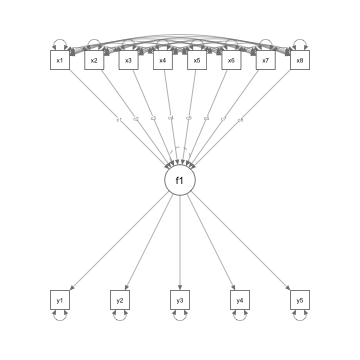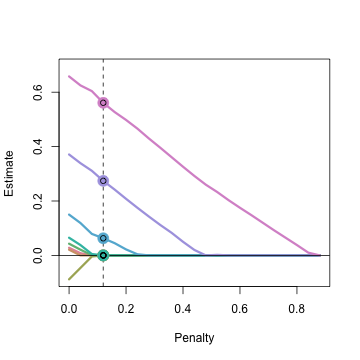``````# install.packages("regsem") # lavaan is a dependency
# install.packages("semPlot")
library(semPlot) # for plotting the model
library(lavaan)
library(regsem)
``````

### Simulate Data

To test out the regsem package, we will first simulate data

``````sim.mod <- "
f1 =~ 1*y1 + 1*y2 + 1*y3+ 1*y4 + 1*y5
f1 ~ 0*x1 + 0*x2 + 0*x3 + 0*x4 + 0*x5 + 0.2*x6 + 0.5*x7 + 0.8*x8
f1~~1*f1"
dat.sim = simulateData(sim.mod,sample.nobs=100,seed=12)
``````

### Run the Model with Lavaan

And then run the model with lavaan so we can better see the structure.

``````run.mod <- "
f1 =~ NA*y1 + y2 + y3+ y4 + y5
f1 ~ c1*x1 + c2*x2 + c3*x3 + c4*x4 + c5*x5 + c6*x6 + c7*x7 + c8*x8
f1~~1*f1
"
lav.out <- sem(run.mod,dat.sim,fixed.x=FALSE)
#summary(lav.out)
parameterestimates(lav.out)[6:13,] # just look at regressions
``````
``````##    lhs op rhs label    est    se      z pvalue ci.lower ci.upper
## 6   f1  ~  x1    c1  0.028 0.119  0.240  0.811   -0.204    0.261
## 7   f1  ~  x2    c2  0.021 0.117  0.179  0.858   -0.208    0.250
## 8   f1  ~  x3    c3 -0.088 0.132 -0.664  0.507   -0.346    0.171
## 9   f1  ~  x4    c4  0.043 0.098  0.438  0.661   -0.149    0.234
## 10  f1  ~  x5    c5  0.065 0.141  0.459  0.646   -0.212    0.342
## 11  f1  ~  x6    c6  0.150 0.123  1.219  0.223   -0.091    0.390
## 12  f1  ~  x7    c7  0.371 0.120  3.104  0.002    0.137    0.606
## 13  f1  ~  x8    c8  0.658 0.133  4.966  0.000    0.399    0.918
``````

### Plot the Model

``````semPaths(lav.out)
``````One of the difficult pieces in using the cv_regsem function is that the penalty has to be calibrated for each particular problem. In running this code, I first tested the default, but this was too small given that there were some parameters > 0.4. After plotting this initial run, I saw that some parameters weren't penalized enough, therefore, I increased the penalty jump to 0.05 and with 30 different values this tested a model (at a high penalty) that had all estimates as zero. In some cases it isn't necessary to test a sequence of penalties that would set “large” parameters to zero, as either the model could fail to converge then, or there is not uncertainty about those parameters inclusion.

``````reg.out <- cv_regsem(lav.out,n.lambda=30,type="lasso",jump=0.04,
pars_pen=c("c1","c2","c3","c4","c5","c6","c7","c8"))
``````

In specifying this model, we use the parameter labels to tell cv_regsem which of the parameters to penalize. Equivalently, we could have used the extractMatrices function to identify which parameters to penalize.

``````# not run
extractMatrices(lav.out)["A"]
``````

Additionally, we can specify which parameters are penalized by type: “regressions”, “loadings”, or both c(“regressions”,“loadings”). Note though that this penalizes all parameters of this type. If you desire to penalize a subset of parameters, use either the parameter name or number format for pars_pen.

Next, we can get a summary of the models tested.

``````summary(reg.out)
``````
``````## CV regsem Object
##  Number of parameters regularized: 8
##  Lambda ranging from 0 to 0.88
##  Lowest Fit Lambda: 0.12
##  Metric: BIC
##  Number Converged: 23
``````

## Plot the parameter trajectories

``````plot(reg.out)
``````Here we can see that we used a large enough penalty to set all parameter estimates to zero. However, the best fitting model came early on, when only a couple parameters were zero.

regsem defaults to using the BIC to choose a final model. This shows up in the final_pars object as well as the lines in the plot. This can be changed with the metric argument.

Understand better what went on with the fit

``````head(reg.out\$fits,10)
``````
``````##       lambda conv   rmsea      BIC    chisq
##  [1,]   0.00    0 0.04584 3954.026 44.69827
##  [2,]   0.04    0 0.04360 3949.874 45.15138
##  [3,]   0.08    0 0.03988 3941.811 46.29903
##  [4,]   0.12    0 0.03580 3933.632 47.32985
##  [5,]   0.16    0 0.03956 3934.808 48.50646
##  [6,]   0.20    0 0.04403 3936.363 50.06142
##  [7,]   0.24    0 0.04874 3938.178 51.87607
##  [8,]   0.28    0 0.05020 3935.423 53.72588
##  [9,]   0.32    0 0.05458 3937.379 55.68232
## [10,]   0.36    0 0.05914 3939.588 57.89149
``````

One thing to check is the “conv” column. This refers to convergence, with 0 meaning the model converged.

And see what the best fitting parameter estimates are.

``````reg.out\$final_pars[1:13] # don't show variances/covariances
``````
``````## f1 -> y1 f1 -> y2 f1 -> y3 f1 -> y4 f1 -> y5 x1 -> f1 x2 -> f1 x3 -> f1
##    0.885    1.093    1.009    1.002    1.021    0.000    0.000    0.000
## x4 -> f1 x5 -> f1 x6 -> f1 x7 -> f1 x8 -> f1
##    0.000    0.000    0.063    0.274    0.561
``````

In this final model, we set the regression paths for x2,x3, x4, and x5 to zero. We make a mistake for x1, but we also correctly identify x6, x7, and x8 as true paths .Maximum likelihood estimation with lavaan had p-values > 0.05 for the parameters simulated as zero, but also did not identify the true path of 0.2 as significant (< 0.05).